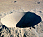xFuzzy set
Posts  1 - 1  of  1Qmars
Hi all
Thank you so much for this useful forum about Fuzzy sets. I want to know if there is any well-known and established method in Fuzzy sets and logic to convert crisp values to interval values? Let me give an example. Let X is a vector with dimension n (called feature vector). I want to convert X into interval (X_lower_bound , X_upper_bound) to use as input patterns for a special type of classifiers that takes interval inputs. Is there any way to do it?
thanks a lot